Скачать презентацию Probability and Information Retrieval Introduction to Artificial Intelligence

• Количество слайдов: 31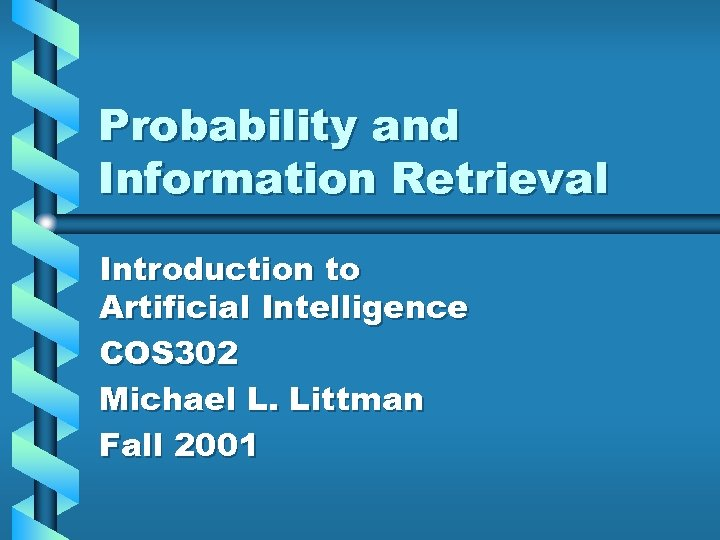Probability and Information Retrieval Introduction to Artificial Intelligence COS 302 Michael L. Littman Fall 2001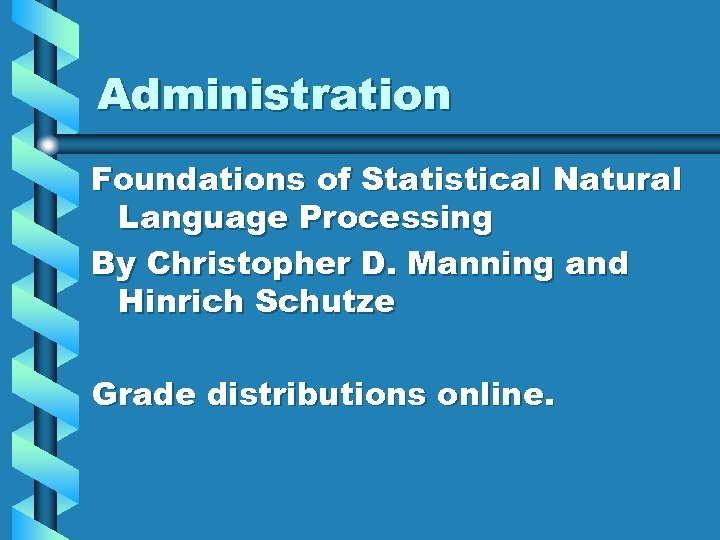Administration Foundations of Statistical Natural Language Processing By Christopher D. Manning and Hinrich Schutze Grade distributions online.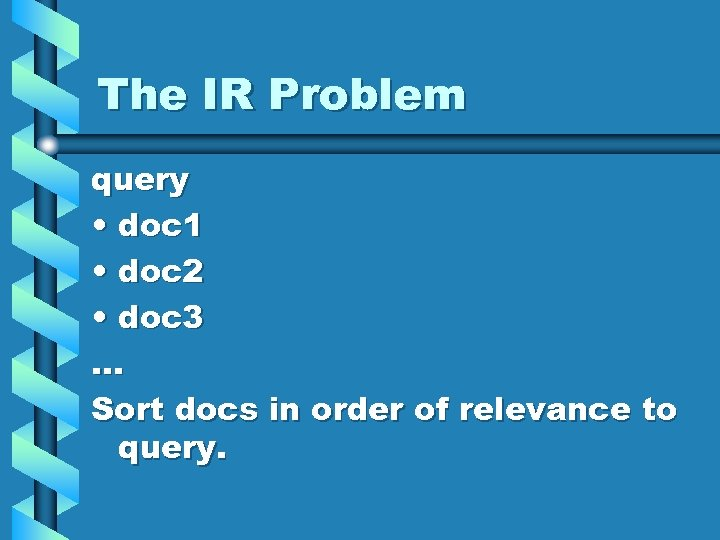The IR Problem query • doc 1 • doc 2 • doc 3. . . Sort docs in order of relevance to query.Example Query: The 1929 World Series 384, 945, 633 results in Alta Vista • GNU's Not Unix! - the GNU Project and the Free Software Foundation (FSF) • Yahoo! Singapore • The USGen. Web Project - Home Page • …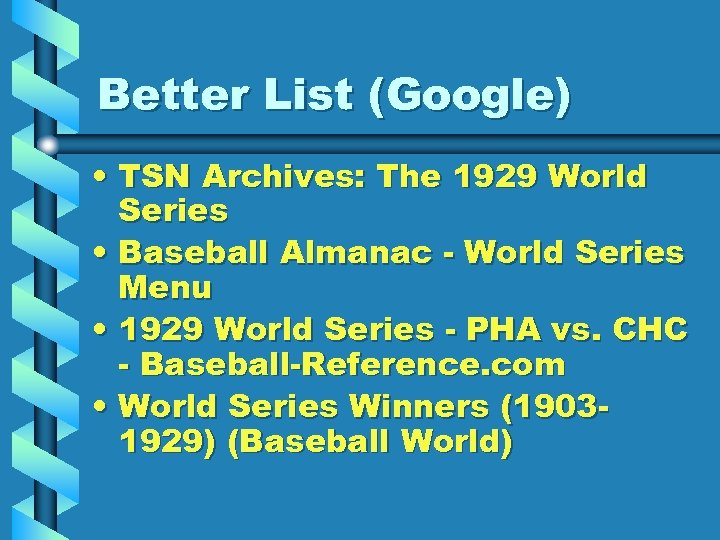Better List (Google) • TSN Archives: The 1929 World Series • Baseball Almanac - World Series Menu • 1929 World Series - PHA vs. CHC - Baseball-Reference. com • World Series Winners (19031929) (Baseball World)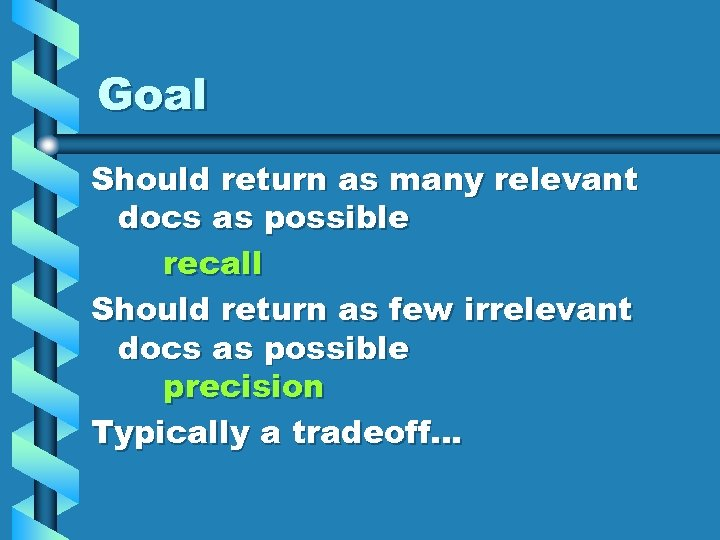Goal Should return as many relevant docs as possible recall Should return as few irrelevant docs as possible precision Typically a tradeoff…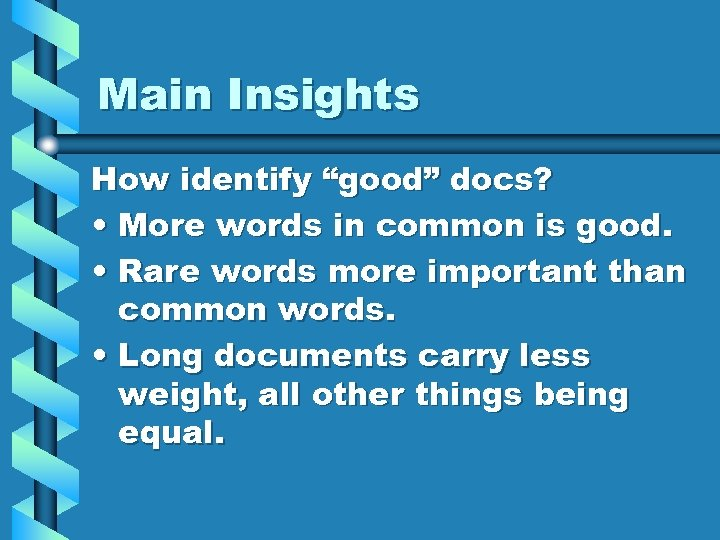Main Insights How identify “good” docs? • More words in common is good. • Rare words more important than common words. • Long documents carry less weight, all other things being equal.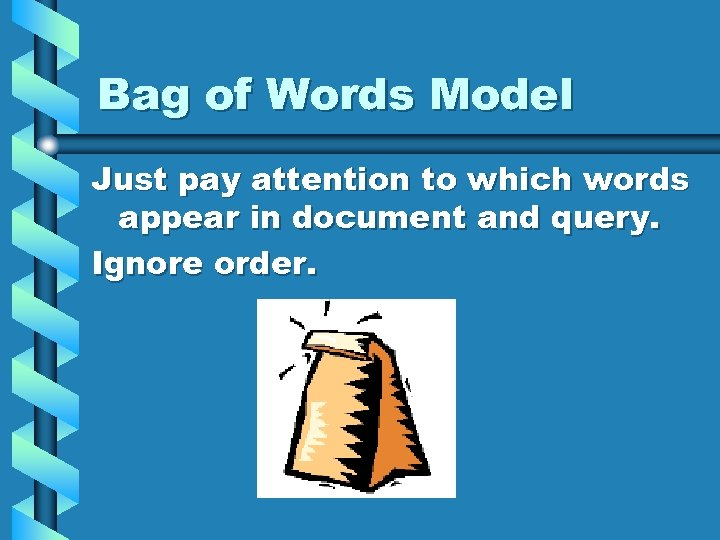Bag of Words Model Just pay attention to which words appear in document and query. Ignore order.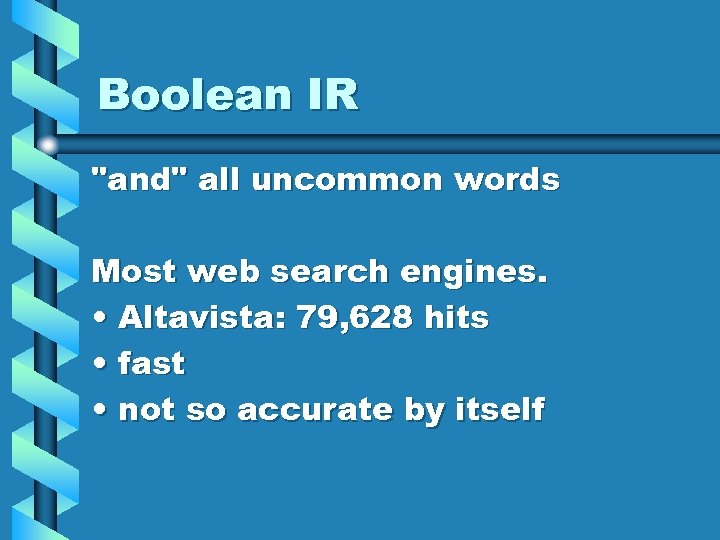Boolean IR "and" all uncommon words Most web search engines. • Altavista: 79, 628 hits • fast • not so accurate by itself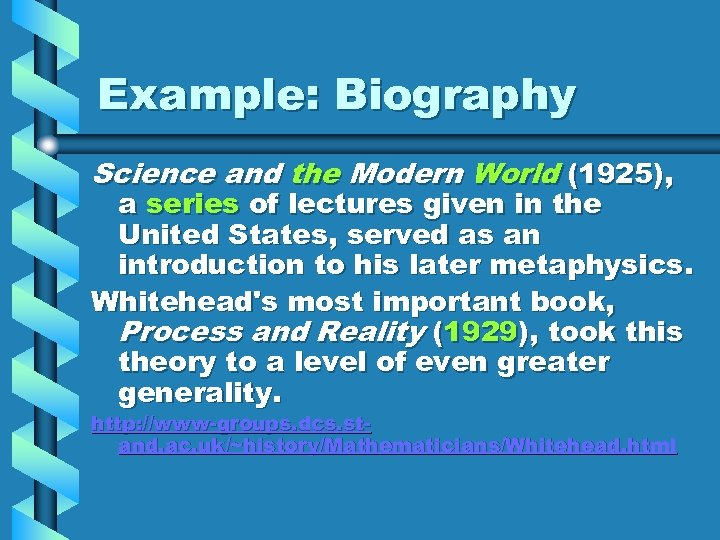Example: Biography Science and the Modern World (1925), a series of lectures given in the United States, served as an introduction to his later metaphysics. Whitehead's most important book, Process and Reality (1929), took this theory to a level of even greater generality. http: //www-groups. dcs. stand. ac. uk/~history/Mathematicians/Whitehead. html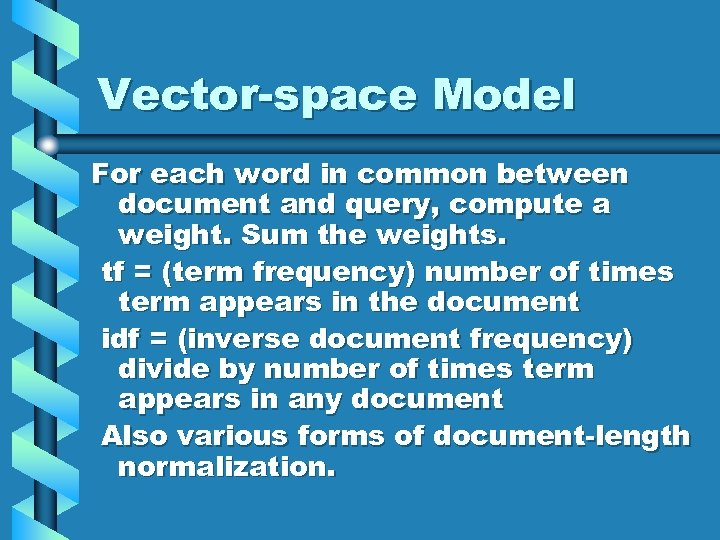Vector-space Model For each word in common between document and query, compute a weight. Sum the weights. tf = (term frequency) number of times term appears in the document idf = (inverse document frequency) divide by number of times term appears in any document Also various forms of document-length normalization.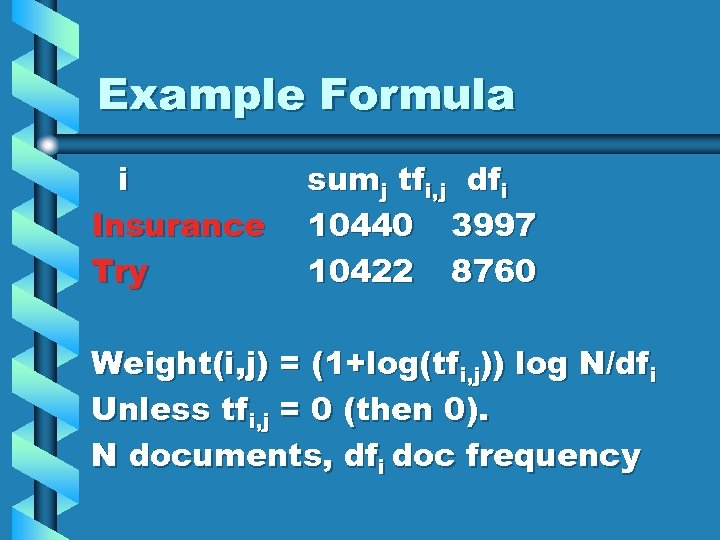Example Formula i Insurance Try sumj tfi, j dfi 10440 3997 10422 8760 Weight(i, j) = (1+log(tfi, j)) log N/dfi Unless tfi, j = 0 (then 0). N documents, dfi doc frequency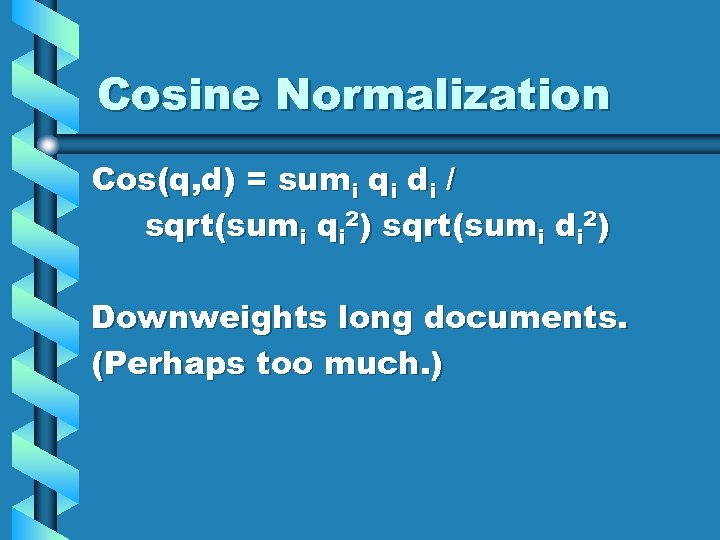Cosine Normalization Cos(q, d) = sumi qi di / sqrt(sumi qi 2) sqrt(sumi di 2) Downweights long documents. (Perhaps too much. )Probabilistic Approach Lots of work studying different weighting schemes. Often very ad hoc, empirically motivated. Is there an analog of A* for IR? Elegant, simple, effective?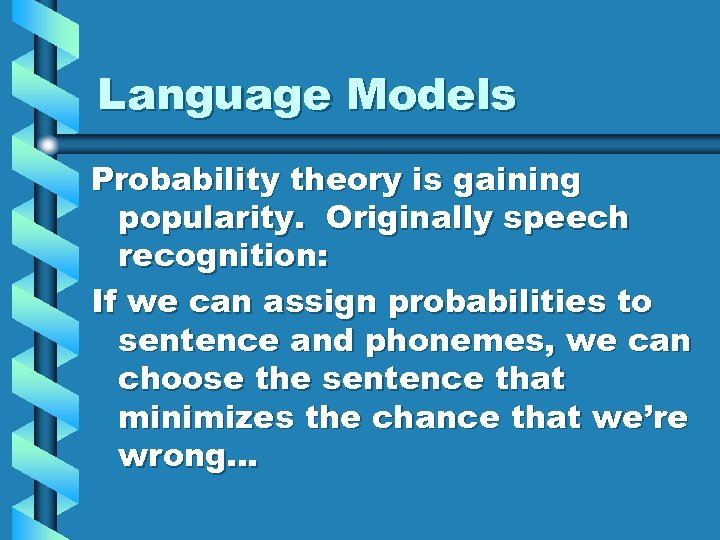Language Models Probability theory is gaining popularity. Originally speech recognition: If we can assign probabilities to sentence and phonemes, we can choose the sentence that minimizes the chance that we’re wrong…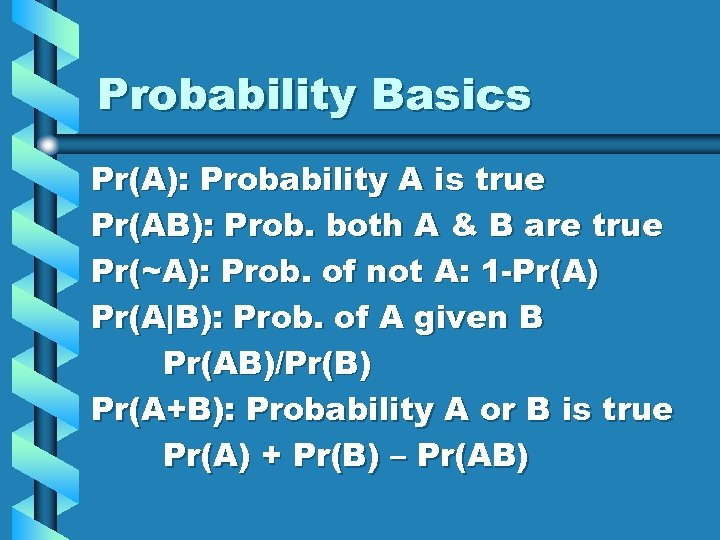Probability Basics Pr(A): Probability A is true Pr(AB): Prob. both A & B are true Pr(~A): Prob. of not A: 1 -Pr(A) Pr(A|B): Prob. of A given B Pr(AB)/Pr(B) Pr(A+B): Probability A or B is true Pr(A) + Pr(B) – Pr(AB)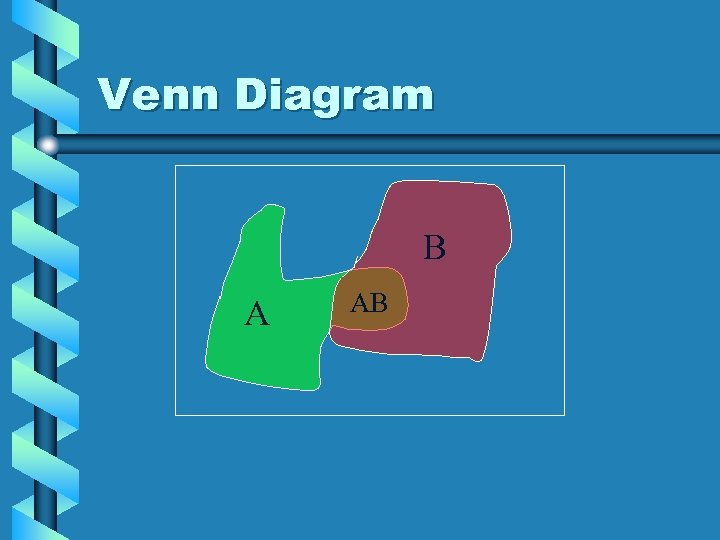Venn Diagram B A AB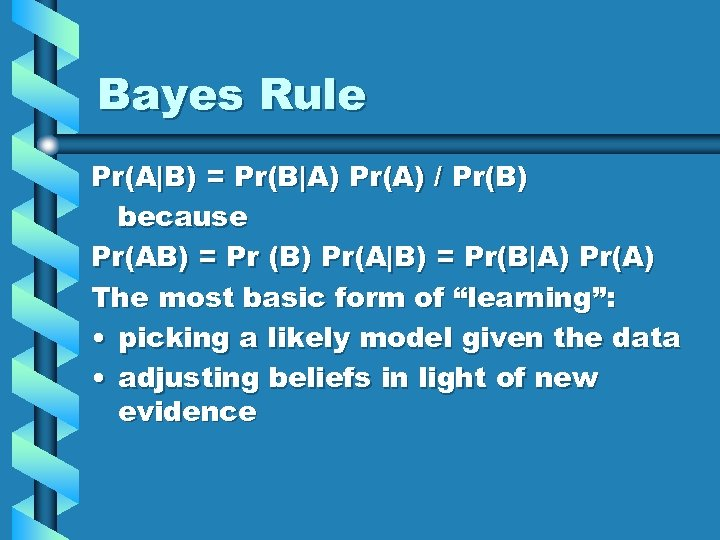Bayes Rule Pr(A|B) = Pr(B|A) Pr(A) / Pr(B) because Pr(AB) = Pr (B) Pr(A|B) = Pr(B|A) Pr(A) The most basic form of “learning”: • picking a likely model given the data • adjusting beliefs in light of new evidence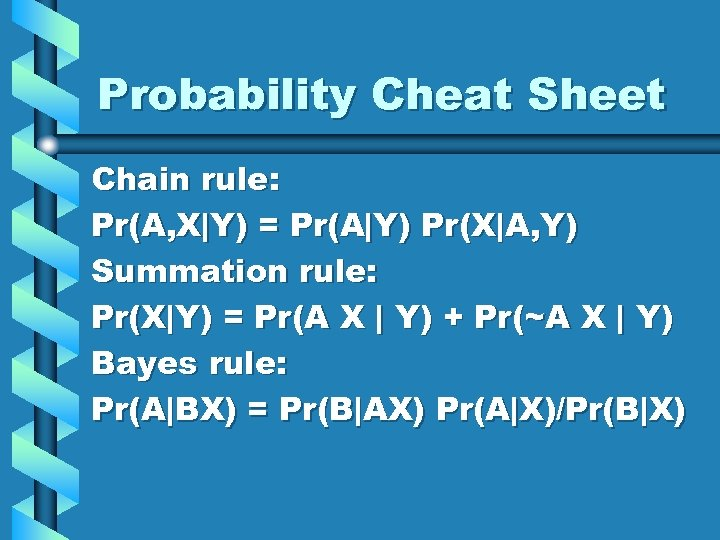Probability Cheat Sheet Chain rule: Pr(A, X|Y) = Pr(A|Y) Pr(X|A, Y) Summation rule: Pr(X|Y) = Pr(A X | Y) + Pr(~A X | Y) Bayes rule: Pr(A|BX) = Pr(B|AX) Pr(A|X)/Pr(B|X)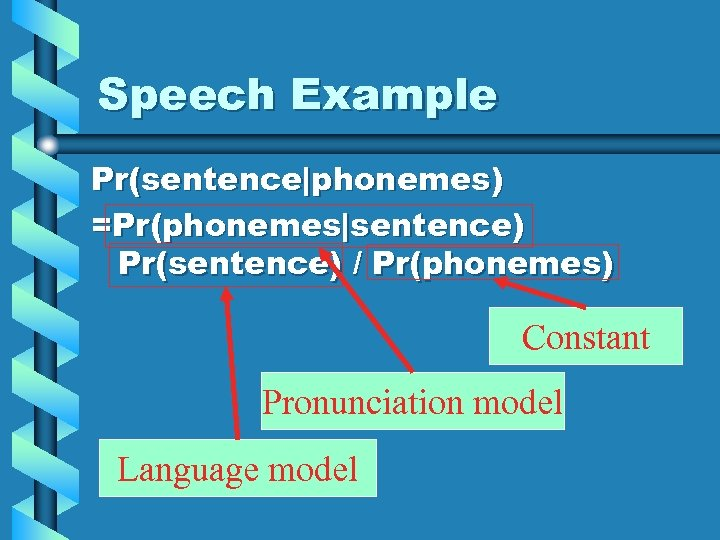Speech Example Pr(sentence|phonemes) =Pr(phonemes|sentence) Pr(sentence) / Pr(phonemes) Constant Pronunciation model Language model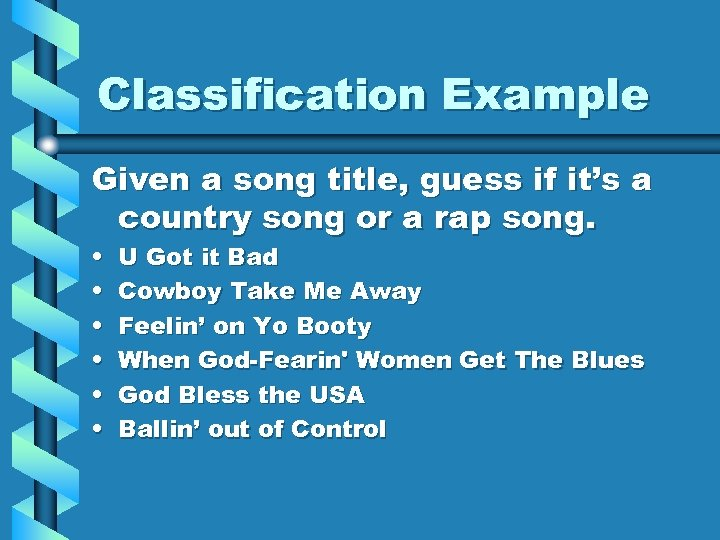Classification Example Given a song title, guess if it’s a country song or a rap song. • • • U Got it Bad Cowboy Take Me Away Feelin’ on Yo Booty When God-Fearin' Women Get The Blues God Bless the USA Ballin’ out of Control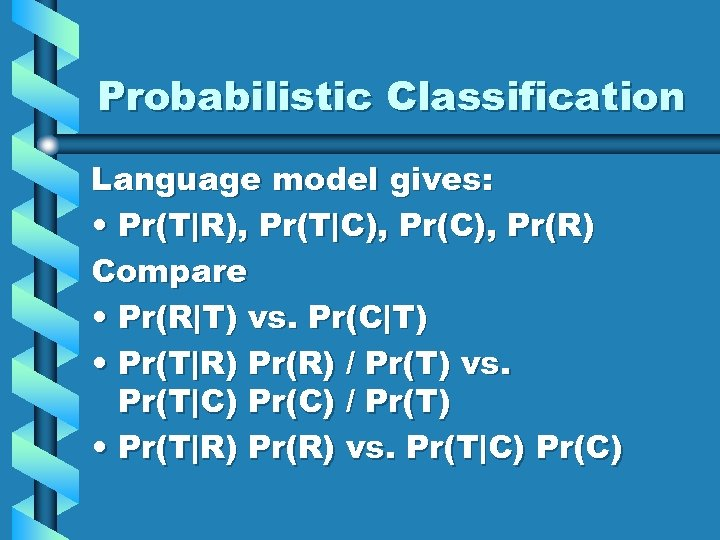Probabilistic Classification Language model gives: • Pr(T|R), Pr(T|C), Pr(R) Compare • Pr(R|T) vs. Pr(C|T) • Pr(T|R) Pr(R) / Pr(T) vs. Pr(T|C) Pr(C) / Pr(T) • Pr(T|R) Pr(R) vs. Pr(T|C) Pr(C)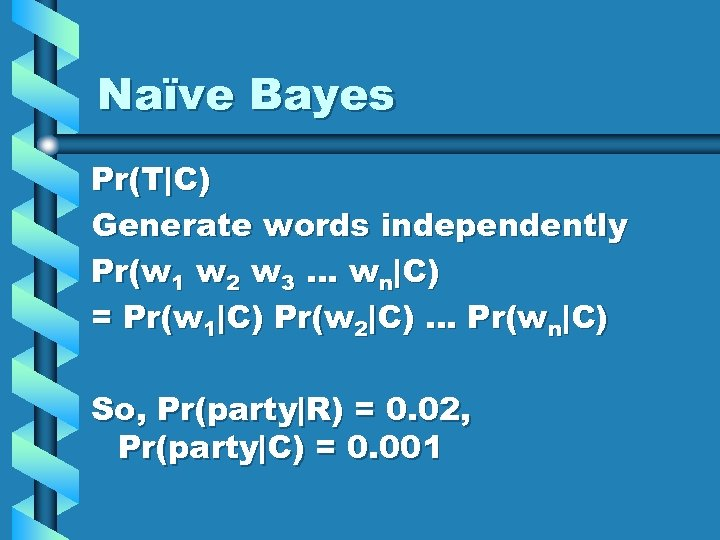Naïve Bayes Pr(T|C) Generate words independently Pr(w 1 w 2 w 3 … wn|C) = Pr(w 1|C) Pr(w 2|C) … Pr(wn|C) So, Pr(party|R) = 0. 02, Pr(party|C) = 0. 001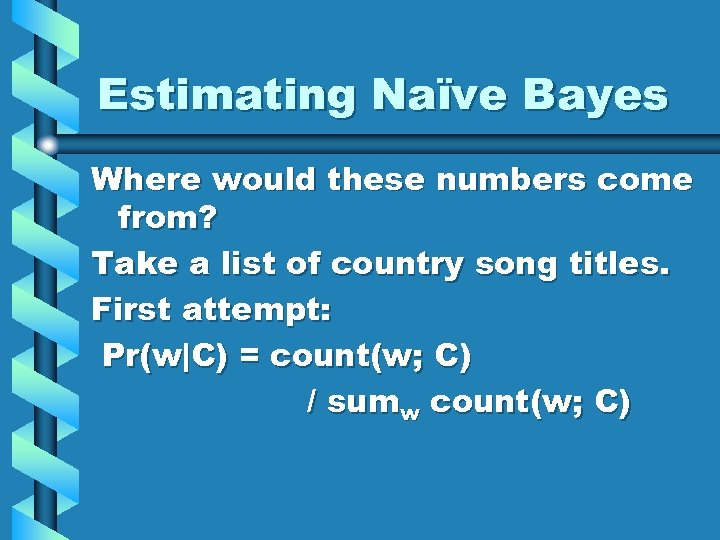Estimating Naïve Bayes Where would these numbers come from? Take a list of country song titles. First attempt: Pr(w|C) = count(w; C) / sumw count(w; C)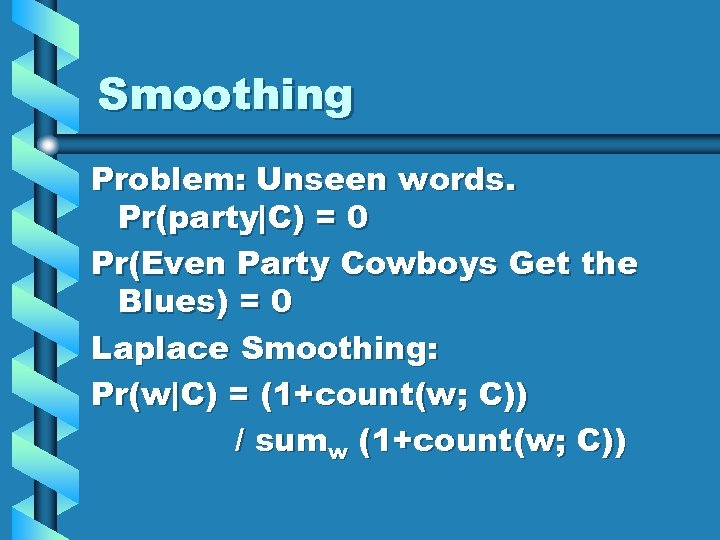Smoothing Problem: Unseen words. Pr(party|C) = 0 Pr(Even Party Cowboys Get the Blues) = 0 Laplace Smoothing: Pr(w|C) = (1+count(w; C)) / sumw (1+count(w; C))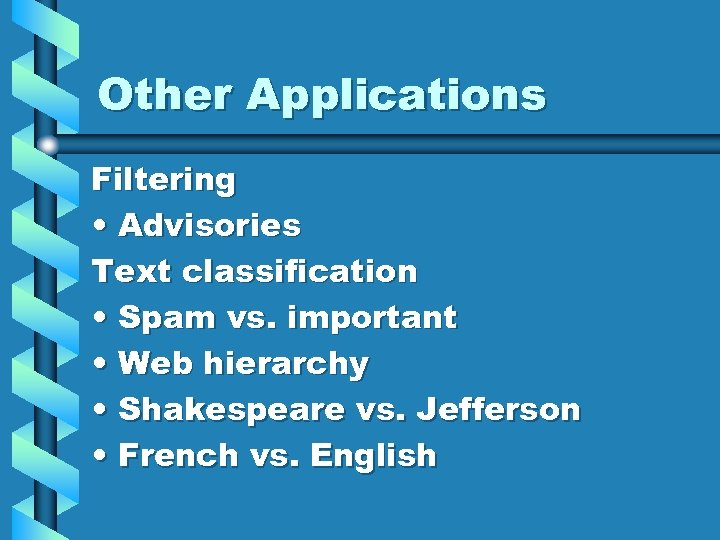Other Applications Filtering • Advisories Text classification • Spam vs. important • Web hierarchy • Shakespeare vs. Jefferson • French vs. English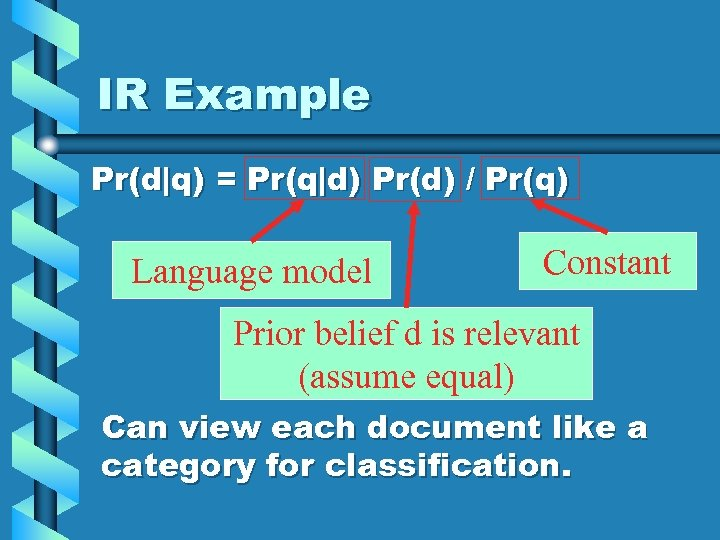IR Example Pr(d|q) = Pr(q|d) Pr(d) / Pr(q) Language model Constant Prior belief d is relevant (assume equal) Can view each document like a category for classification.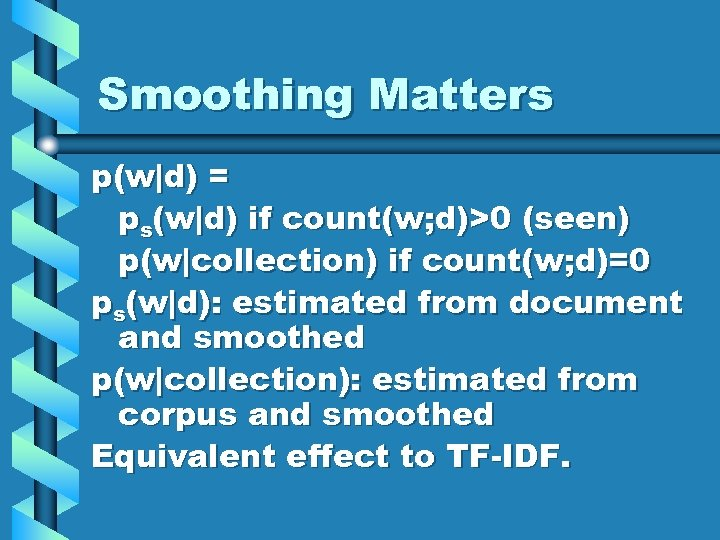Smoothing Matters p(w|d) = ps(w|d) if count(w; d)>0 (seen) p(w|collection) if count(w; d)=0 ps(w|d): estimated from document and smoothed p(w|collection): estimated from corpus and smoothed Equivalent effect to TF-IDF.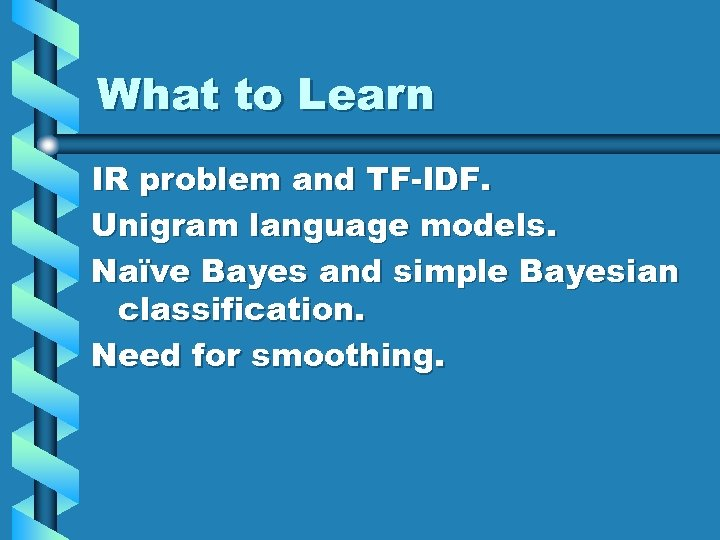What to Learn IR problem and TF-IDF. Unigram language models. Naïve Bayes and simple Bayesian classification. Need for smoothing.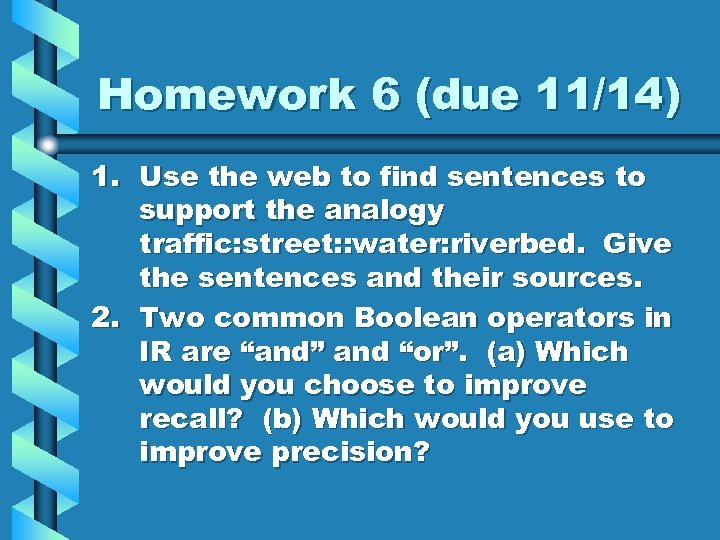Homework 6 (due 11/14) 1. Use the web to find sentences to support the analogy traffic: street: : water: riverbed. Give the sentences and their sources. 2. Two common Boolean operators in IR are “and” and “or”. (a) Which would you choose to improve recall? (b) Which would you use to improve precision?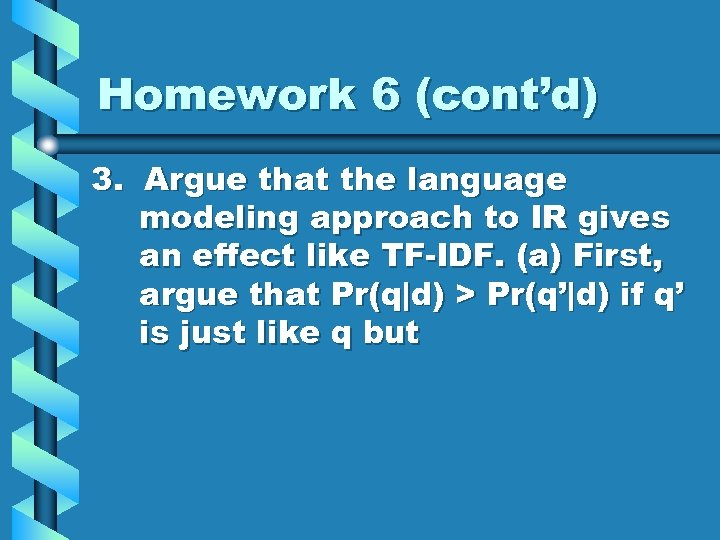Homework 6 (cont’d) 3. Argue that the language modeling approach to IR gives an effect like TF-IDF. (a) First, argue that Pr(q|d) > Pr(q’|d) if q’ is just like q but